# E-PolyLearning

 1. In the following question, you are given a figure (X) followed by four alternative figures (1), (2), (3) and (4) such that figure (X) is embedded in one of them. Find out the alternative figure which contains figure (X) as its part.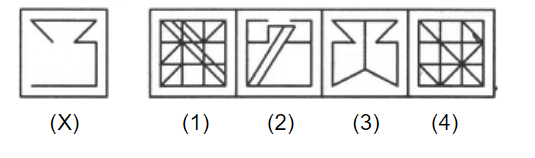a. 1 b. 2 c. 3 d. 4

 2. In the following question, you are given a figure (X) followed by four alternative figures (1), (2), (3) and (4) such that figure (X) is embedded in one of them. Find out the alternative figure which contains figure (X) as its part.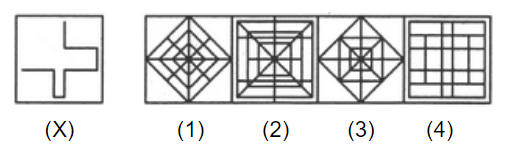a. 1 b. 2 c. 3 d. 4
 3. In the following question, you are given a figure (X) followed by four alternative figures (1), (2), (3) and (4) such that figure (X) is embedded in one of them. Find out the alternative figure which contains figure (X) as its part.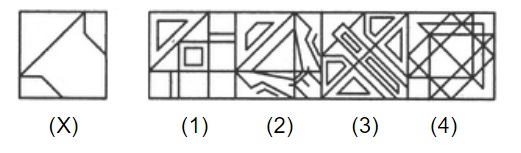a. 1 b. 2 c. 3 d. 4
 4. In the following question, you are given a figure (X) followed by four alternative figures (1), (2), (3) and (4) such that figure (X) is embedded in one of them. Find out the alternative figure which contains figure (X) as its part.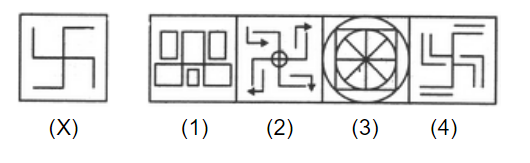a. 1 b. 2 c. 3 d. 4
 5. In the following question, you are given a figure (X) followed by four alternative figures (1), (2), (3) and (4) such that figure (X) is embedded in one of them. Find out the alternative figure which contains figure (X) as its part.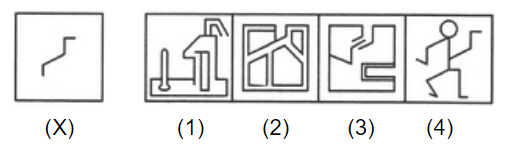a. 1 b. 2 c. 3 d. 4